## binary digit sum mod 3

October 21, 2012
Problem: For length n bit strings, x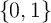n = x0x1xn-1, define digitsum(x) = ixi as the number of 1s in x. We want to know the number of bit strings that have digitsum(x) 0( mod3).

For 0 k n, the number of bit strings with digitsum(x) = k is given by the binomial co-efficient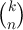. Thus the count we want is given byConsider the primitive cube root of 1, ω = -+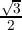i. Since ω2 = -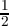-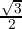i and ω3 = 1, we have the real part (ωk) = 1 if k 0( mod3) and -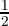otherwise. We can scale this to get a function of k which is 1 for k divisible by 3 and 0 otherwise, i.e. with f(k) =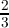(ωk) +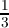, we haveThus, the count isNow, 1 + ω =+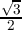is the primitive sixth root of 1, and we have (1 + ω)n = 1,,-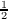,-1,-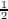,respectively, based on n( mod3) 0, 1, 2, 3, 4, 5. Substituting in the above equation, our count =±or. The counts for digitsum(x) 1 or 2( mod3) is similar except that we use ωk+2 or ωk+1 in the equation. This gives us the final table:

 n mod3 0 1 2 3 4 5 count(digitsum(x) ≡ 0( mod3)) =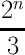+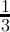-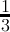-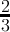-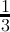count(digitsum(x) ≡ 1( mod3)) =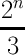+ -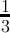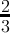-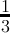-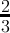count(digitsum(x) ≡ 2( mod3)) =+ -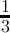-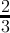-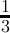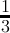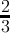Anil Das